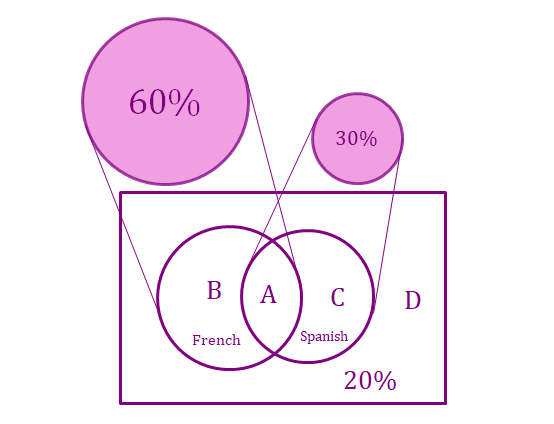# Probability Quiz Seems Incorrect, Explanation Doesn't Make Sense

One of the probability quizzes asks:

A high school offers courses in French and in Spanish (and only those two languages). A sample of students from the school were asked which language courses they enrolled in. 60% of the students responded that they took French, 30% of the students responded that they took Spanish, and 20% of the students responded that they did not enroll in any language course.

If a student is randomly chosen from among the students surveyed, what is the probability that the student enrolled in the French course, but not in Spanish course?

My thought was that the answer would be 52%, but they disagree:

Correct answer: 0.5

If 20% of students are not in a language course, then 80% of students are in a language course. 60% are in French and 30% are in Spanish, so that means that 10% must be enrolled in both. Then 50% of students are enrolled in the French course, but not the Spanish course. Thus, the probability that a student would be enrolled in the French course, but not the Spanish course, is 0.5

1. I don't understand how they get that 10% number based on their explanation
2. Where do they get the 50% number?

My thinking is that there's no way to tell, because it's possible that all 30% of the Spanish students are also French students.

Or, if they mean those surveyed responded that they took ONLY Spanish or ONLY French, then that would mean there's a 60% chance to be a French student and a 30% chance to be a Spanish student, and the chances of being ONLY a French OR Spanish student would be 60% + 30% - 60*30% which is 72%, leaving 8% of the 80% of students taking a foreign language to take both languages. So, students taking french (60%) minus students taking both (8%) is 52%.Note by Byron Altice
2 years, 10 months ago

This discussion board is a place to discuss our Daily Challenges and the math and science related to those challenges. Explanations are more than just a solution — they should explain the steps and thinking strategies that you used to obtain the solution. Comments should further the discussion of math and science.

When posting on Brilliant:

• Use the emojis to react to an explanation, whether you're congratulating a job well done , or just really confused .
• Ask specific questions about the challenge or the steps in somebody's explanation. Well-posed questions can add a lot to the discussion, but posting "I don't understand!" doesn't help anyone.
• Try to contribute something new to the discussion, whether it is an extension, generalization or other idea related to the challenge.
• Stay on topic — we're all here to learn more about math and science, not to hear about your favorite get-rich-quick scheme or current world events.

MarkdownAppears as
*italics* or _italics_ italics
**bold** or __bold__ bold
- bulleted- list
• bulleted
• list
1. numbered2. list
1. numbered
2. list
Note: you must add a full line of space before and after lists for them to show up correctly
paragraph 1paragraph 2

paragraph 1

paragraph 2

[example link](https://brilliant.org)example link
> This is a quote
This is a quote
    # I indented these lines
# 4 spaces, and now they show
# up as a code block.

print "hello world"
# I indented these lines
# 4 spaces, and now they show
# up as a code block.

print "hello world"
MathAppears as
Remember to wrap math in $$ ... $$ or $ ... $ to ensure proper formatting.
2 \times 3 $2 \times 3$
2^{34} $2^{34}$
a_{i-1} $a_{i-1}$
\frac{2}{3} $\frac{2}{3}$
\sqrt{2} $\sqrt{2}$
\sum_{i=1}^3 $\sum_{i=1}^3$
\sin \theta $\sin \theta$
\boxed{123} $\boxed{123}$

## Comments

Sort by:

Top Newest

If you consider all possibilities, there will be only 4 types of students:

(A) Those who took French and Spanish

(B) Those who took only French

(C) Those who took only Spanish

(D) Those who took neither

Since any student you pick must fall into one of the above categories, that means if you add up all the percentages of these 4 types of students, you must get 100% (surprise!).

The problem states that 60% of students took French (the problem does not say anything about whether these 60% of people took Spanish or not), we conclude that $P(A)+P(B)=60\%$.

Then it states that 30% of students took Spanish (again this does not say anything about whether they took French), we conclude that $P(A)+P(C)=30\%$.

20% took neither French nor Spanish, $P(D)=20\%$.

Now add up all the equations we have so far, we have $2P(A)+P(B)+P(C)+P(D)=110\%$, but we know that $P(A)+P(B)+P(C)+P(D)=100\%$, thus we have $P(A)=10\%$ i.e. 10% of students took French and Spanish.

Therefore, the percentage (probability) of students who took French but not Spanish is $P(B)=60\%-P(A)=60\%-10\%=50\%$. $_\square$

This problem can also be tackled visually using Venn diagrams:Since everything must add up to 100%, if we just add up the percentages, we get 110%. Where did the extra 10% come from? It's the overlapping region between the circle of students who took French and the circle of students who took Spanish. Therefore $P(B)=60\%-10\%=50\%$.

I hope this clears your confusion. :)

- 2 years, 10 months ago

Log in to reply

Yes, great explanation, it makes sense now, thank you

- 2 years, 10 months ago

Log in to reply

×

Problem Loading...

Note Loading...

Set Loading...